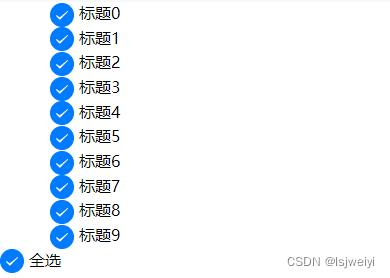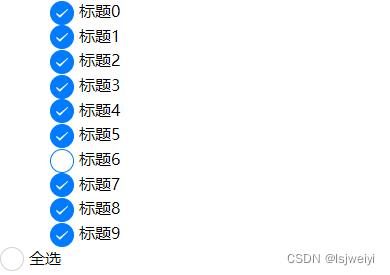﻿ uniapp多选框全选功能的实现思路与方法实例 - 自由资讯

# uniapp多选框全选功能的实现思路与方法实例

• 前言
• 实现思路
• 总结

## 前言

uniapp内置的`checkbox`其实以及`checkbox-group`本来挺好的，但是有两个问题：

• 无法依赖其事件实现全选
• 样式固定，难以修改

## 实现思路

```<script setup lang="ts">
import { reactive } from "vue";
// 模拟的数据对象，要是响应式的
let data = reactive([] as { id: number; text: string; selected: boolean }[]);
// 生成数据
for (let i = 0; i < 10; i++) {
data.push({
id: i + 5,
text: "标题" + i,
selected: false,
});
}
</script>
```

```    // 存储已选内容， 因为这个列表是增删很频繁的，所以选用map而不是数组，key对应的是数据的下标。至于value存放什么，完全由自己定
let selected = reactive(new Map<number, number>());
```

```    // 选项框点击事件，参数是数据的下标
function checkbox(index: number) {
// 选中的状态下再次点击，即为取消选中
if (data[index].selected) {
data[index].selected = false;
selected.delete(index); // 然后删除对应key即可
}
// 未选中状态下点击
else {
data[index].selected = true;
selected.set(index, data[index].id);
}
}
```

```    // 全选与反选事件
function allSelect() {
// 已经全选情况下，就是反选，全选就说明长度一样
if (selected.size === data.length) {
selected.clear(); // 全部清除
data.forEach((element) => {
element.selected = false; // 全部不选，就行了
});
}
// 还未全选的状态下
else {
data.forEach((element, index) => {
// 因为可能存在部分已经选择了，所以得先判断是否已存在，不存在才需要添加
if (!selected.has(index)) {
selected.set(index, element.id); // key是对应的下标index，而value是可以自定义的
element.selected = true; // 设为选中
}
});
}
}
```

```<template>
<!-- 是个多选列表 -->
<view v-for="(item, index) in data">
<label style="margin-left: 50px">
<radio :value="String(index)" :checked="item.selected" @click="checkbox(index)" />{{ item.text }}
</label>
</view>
<!-- 全选按钮 -->
<label> <radio value="1" :checked="selected.size === data.length" @click="allSelect" />全选</label>
</template>
```

```<template>
<!-- 是个多选列表 -->
<view v-for="(item, index) in data">
<label style="margin-left: 50px">
<radio :value="String(index)" :checked="item.selected" @click="checkbox(index)" />{{ item.text }}
</label>
</view>
<!-- 全选按钮 -->
<label> <radio value="1" :checked="selected.size === data.length" @click="allSelect" />全选</label>
</template>
<script setup lang="ts">
import { reactive } from "vue";

// 模拟的数据对象，要是响应式的
let data = reactive([] as { id: number; text: string; selected: boolean }[]);
// 生成数据
for (let i = 0; i < 10; i++) {
data.push({
id: i + 5,
text: "标题" + i,
selected: false,
});
}

// 存储已选内容， 因为这个列表是增删很频繁的，所以选用map而不是数组，key对应的是数据的下标。至于value存放什么，完全由自己定
let selected = reactive(new Map<number, number>());

// 全选与反选事件
function allSelect() {
// 已经全选情况下，就是反选，全选就说明长度一样
if (selected.size === data.length) {
selected.clear(); // 全部清除
data.forEach((element) => {
element.selected = false; // 全部不选，就行了
});
}
// 还未全选的状态下
else {
data.forEach((element, index) => {
// 因为可能存在部分已经选择了，所以得先判断是否已存在，不存在才需要添加
if (!selected.has(index)) {
selected.set(index, element.id); // key是对应的下标index，而value是可以自定义的
element.selected = true; // 设为选中
}
});
}
}

// 选项框点击事件，参数是数据的下标
function checkbox(index: number) {
// 选中的状态下再次点击，即为取消选中
if (data[index].selected) {
data[index].selected = false;
selected.delete(index); // 然后删除对应key即可
}
// 未选中状态下点击
else {
data[index].selected = true;
selected.set(index, data[index].id);
}
}
</script>
<style></style>```﻿